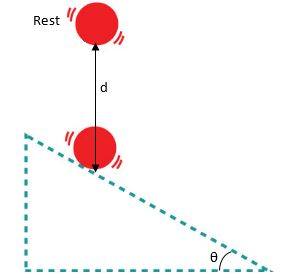# Ball Falling On Inclined RampFrom a resting state, a small ball falls vertically on an inclined ramp of angle $\theta$, in respect to the horizontal, resulting in several elastic collisions.

If $d$ is the initial vertical distance from the ball to the ramp, find the distance between the $n^{\text{th}}$ point of collision and the first point of collision in a function of $d$, $n$ and $\theta.$

This question appeared on ITA's 2014 Physics Paper, and is inspired by a question from Saraeva's book "Problemas Selecionados de Fisica Elementar".

×

Problem Loading...

Note Loading...

Set Loading...